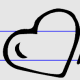Directions: Fill in the blanks with numbers that make the equation true.

## 4 + 5 = ___ – ___

### Hint

If I cover the right side of the equation, what is 4 + 5 the same as?  So, how much more do we need on the right side to make it the same as 4 + 5?

Many possibilities. As long as the difference is 9.
Some of the solutions are:
10-1,

9-0,

12-3,

20-11
and so on…

### Extension

How many different sets of numbers can go in there?

Source: Nanette Johnson

### Check Also

Directions: Using the digits 0 to 9 at most one time each, place a digit …

1.I don’t see how this is an “open middle” question. Wouldn’t it be more interesting to ask the child how many ways can we make this equation true (4+5= _ + _ )? What if we changed the “+” on the right to a “-” ? Then how many answers could we get? These questions could really get kids thinking.

•Great suggestion. I made the change. Thank you for making the problem better.

2.I really liked the problem as it was for my 1st grade daughter- it let her develop a different understanding of the equal sign, and with a variety of examples tried she demonstrated her thinking in different ways which grew in sophistication.

3.4.no it does not

5.